## Statement

The set of nilpotent elements in a commutative unital ring is an ideal (this ideal is termed the nilradical).

## Proof

Commutativity is crucial to the proof, as we shall see.

### Abelian group structure

It is clear that$0$ is nilpotent, and that if$x$ is nilpotent, so is$-x$. We thus only need to show closure under addition. Suppose$x$ and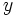$y$ are nilpotent with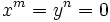$x^m = y^n = 0$. Then consider: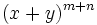$(x+y)^{m+n}$

We can expand this by the binomial theorem. We get a sum of monomials. For each monomial, either the power of$x$ is at least$m$ or the power of$y$ is at least$n$. Thus, each of the monomials in the expansion is zero, and so the above expression simplifies to 0.

Commutativity is essentially to rewrite expressions like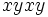$xyxy$ as a power of$x$, times a power of$y$.

### The ideal property

We need to show that if$x$ is nilpotent, and$a$ is any ring element, then$ax$ is nilpotent. Since there exists a$n$ such that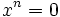$x^n = 0$, we have$(ax)^n = a^nx^n = 0$. Here, we again use commutativity to move the$a$s past the$x$s.